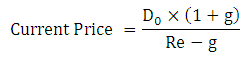# Babbalu Inc. just paid a dividend of \$1 per share. Babbalu expects to increase its annual dividend by 20% per year for the next two years and by 15% per year for the following two years. Starting in year 5, Babbalu is expects to pay a constant annual dividend of \$3 a share. What is the current value of this stock if the required rate of return is 12%?

Question
1 views

Babbalu Inc. just paid a dividend of \$1 per share. Babbalu expects to increase its annual dividend by 20% per year for the next two years and by 15% per year for the following two years. Starting in year 5, Babbalu is expects to pay a constant annual dividend of \$3 a share. What is the current value of this stock if the required rate of return is 12%?

check_circle

Introduction

Current value of share can be calculated by computing the present value of all the future dividends.

Present value of future dividends/current market price can be calculated by using the following formula:Here,

Current dividend is "D0"

Growth rate is "g"

Requir...

### Want to see the full answer?

See Solution

#### Want to see this answer and more?

Solutions are written by subject experts who are available 24/7. Questions are typically answered within 1 hour.*

See Solution
*Response times may vary by subject and question.
Tagged in